International Tables for Crystallography (2010). Vol. B, ch. 1.5, pp. 175-192   | 1 | 2 | https://doi.org/10.1107/97809553602060000762

Contents

• 1.5. Crystallographic viewpoints in the classification of space-group representations  (pp. 175-192)
• 1.5.1. List of abbreviations and symbols  (p. 175) | html | pdf |
• 1.5.2. Introduction  (pp. 175-176) | html | pdf |
• 1.5.3. Basic concepts  (pp. 176-179) | html | pdf |
• 1.5.3.1. Representations of finite groups  (p. 176) | html | pdf |
• 1.5.3.2. Space groups  (pp. 176-177) | html | pdf |
• 1.5.3.3. Representations of the translation groupand the reciprocal lattice  (pp. 177-178) | html | pdf |
• 1.5.3.4. Irreducible representations of space groups and the reciprocal-space group  (pp. 178-179) | html | pdf |
• 1.5.4. Conventions in the classification of space-group irreps  (pp. 179-181) | html | pdf |
• 1.5.4.1. Fundamental regions  (p. 179) | html | pdf |
• 1.5.4.2. Minimal domains  (pp. 179-180) | html | pdf |
• 1.5.4.3. Wintgen positions  (pp. 180-181) | html | pdf |
• 1.5.5. Examples and discussion  (pp. 181-191) | html | pdf |
• 1.5.5.1. Guide to the figures  (pp. 182-184) | html | pdf |
• 1.5.5.2. Guide to the k -vector tables  (pp. 184-188) | html | pdf |
• 1.5.5.3. Figures and tables  (pp. 188-189) | html | pdf |
• 1.5.5.4. Discussion  (pp. 189-191) | html | pdf |
• 1.5.5.4.1. Representation domains and asymmetric units  (pp. 189-190) | html | pdf |
• 1.5.5.4.2. Splitting of k -vector types  (p. 190) | html | pdf |
• 1.5.5.4.3. k -vector types for non-holosymmetric space groups  (p. 190) | html | pdf |
• 1.5.5.4.4. Ranges of independent parameters  (pp. 190-191) | html | pdf |
• 1.5.6. Conclusions  (p. 191) | html | pdf |
• Appendix 1.5.1. Reciprocal-space groups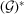(p. 192) | html | pdf |
• References | html | pdf |
• Figures
• Fig. 1.5.5.1. Brillouin zone with asymmetric unit and representation domain of CDML for arithmetic crystal class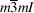(p. 183) | html | pdf |
• Fig. 1.5.5.2. Brillouin zone with asymmetric unit and representation domain of CDML for arithmetic crystal class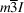(p. 184) | html | pdf |
• Fig. 1.5.5.3. Brillouin zone with asymmetric unit and representation domain of CDML for arithmetic crystal class: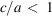(p. 185) | html | pdf |
• Fig. 1.5.5.4. Brillouin zone with asymmetric unit and representation domain of CDML for arithmetic crystal class: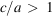(p. 186) | html | pdf |
• Fig. 1.5.5.5. Brillouin zone with asymmetric unit and representation domain of CDML for arithmetic crystal class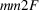: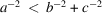,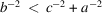and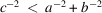(p. 187) | html | pdf |
• Fig. 1.5.5.6. Brillouin zone with asymmetric unit and representation domain of CDML for arithmetic crystal class: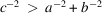(p. 188) | html | pdf |
• Fig. 1.5.5.7. Brillouin zone with asymmetric unit and representation domain of CDML for arithmetic crystal class: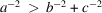(p. 189) | html | pdf |
• Tables
• Table 1.5.4.1. Conventional coefficientsof k expressed by the adjusted coefficientsof IT A for the different Bravais types of lattices in direct space  (p. 181) | html | pdf |
• Table 1.5.4.2. Primitive coefficientsof k from CDML expressed by the adjusted coefficientsof IT A for the different Bravais types of lattices in direct space  (p. 181) | html | pdf |
• Table 1.5.5.1. List of k -vector types for arithmetic crystal class(p. 183) | html | pdf |
• Table 1.5.5.2. List of k -vector types for arithmetic crystal class(p. 184) | html | pdf |
• Table 1.5.5.3. List of k -vector types for arithmetic crystal class:(p. 185) | html | pdf |
• Table 1.5.5.4. List of k -vector types for arithmetic crystal class:(p. 186) | html | pdf |
• Table 1.5.5.5. List of k -vector types for arithmetic crystal class:,and(p. 187) | html | pdf |
• Table 1.5.5.6. List of k -vector types for arithmetic crystal class: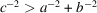(p. 188) | html | pdf |
• Table 1.5.5.7. List of k -vector types for arithmetic crystal class:(p. 189) | html | pdf |